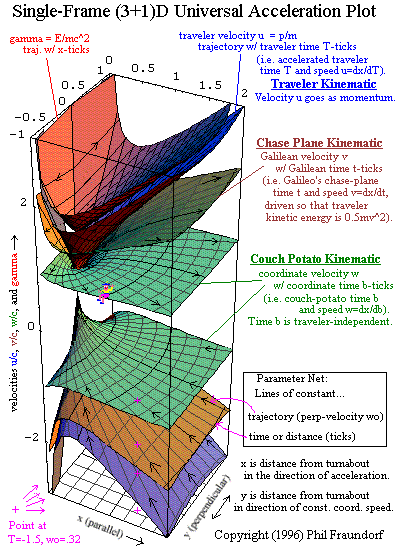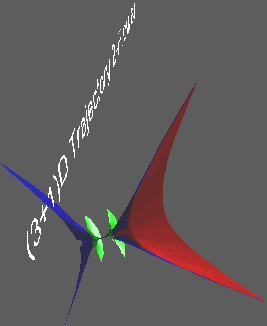# Single-Frame (3+1)D Universal Acceleration Plot

• From notes on "Introducing Newcomers to the 21st Century"
• Copyright 1995-96 by Phil Fraundorf, Dept. of Physics & Astronomy
• University of Missouri-StL, St. Louis MO 63121-4499
• Phone: (314)516-5044, Fax:(314)516-6152
• At UM-StLouis see also: accel1, cme, programs, stei-lab, & wuzzlers.
• A table of contents for these "frame-dependent relativity" pages.
• For source, cite URL at http://www.umsl.edu/~fraundor/univpl3d.html
• Version release date: 21 June 1996.
• Mindquilts site page requests ~2000/day approaching a million per year.
• Requests for a "stat-counter linked subset of pages" since 4/7/2005: .

• As in the other pre-transform relativity stuff described here, if one measures all distances with respect to one inertial frame but defines several velocities, then relativistic acceleration and time dilation effects can be dealt with both graphically and quantitatively before multiple frames and its complications need be considered. These several velocities are the usual coordinate velocity v, plus proper velocity w or distance per unit traveler time, and Galilean velocity V corresponding distance traveled per unit time for an observer defining time so that kinetic energy is 0.5mV^2. Note: The symbols used on the plot differ from the more conventional ones used in this paragraph.

If we plot displacement x in a direction parallel to the coordinate acceleration, and displacement y in whatever direction our accelerated object is moving perpendicular to x, then by putting everything in suitably dimensionless units*, all times and velocities (plus energy) can be plotted as a function of position in this frame, with a single surface per variable on which all constant acceleration problems in (3+1)D special relativity plot! The graph below introduces you to some of those surfaces.To enhance viewability, instead of plotting coordinate, Galilean and proper times in addition to E/mc^2 and all three velocities, we've provided information on the passage of time along each trajectory with the help of "tick marks" with which the various trajectory surfaces are parameterized. We've attempted to space the tick marks by 0.25 in the appropriate dimensionless units. However, a comparitive view of time surfaces as well is constructive (cf. the 1+1D examples elsewhere on these pages), as is a comparable view of Galilean constant acceleration set in a Galilean (rather than a Lorentz or Minkowski) space time. After all, most of the accelerations humans have encountered in history plot in the stem of this flower, where the Galilean surface is indistinguishable from the other two! These will be linked hereto, when we get the chance.

Since we have plotted velocity magnitudes which are always greater than zero, but wanted to provide surfaces both for incoming and outgoing motion (relative to the rest event), the upward sequence of velocity surfaces is repeated in the downward direction as well. This means that when an incoming traveler hits the y=0 plane (this is also the 1+1D universal plot), they switch instantly by definition to the companion outgoing surface. If anyone wants to put together some animations in this regard, for example in conjuction with the solution of specific types of constant acceleration problems, by all means let me know!

*To make the units "dimensionless", plot distances in units of c^2 over the proper acceleration ao, times in units of c/ao, and velocities in units of lightspeed c.

For those who might like a more interactive look at this naturally occurring "trajectory flower", we've put one such flower into a 380kB VRML file linked to the image below. If you have a viewer for such files, like Netscape Navigator 2.0 with Live3D, then you can spin, and zoom around through, the structure as you wish. Here is a link to a javaview translation of the same model.The section of the universal plot provided here covers trajectories for coordinate velocity (green) during map times from -2 to +2, and proper velocity (blue) during proper times from -2 to +2, with all times measured relative to the rest event in units of c/ao (or years at 1 gee). Values of transverse coordinate velocity (a constant for a given trajectory) range from -0.9c to 0.9c (i.e. around 2 lightyears/traveler year). There is also a red surface representing E/mc^2 for the x-distance traveled relative to rest of 0 to +2, in units of c^2/ao (or lightyears at 1 gee). See if you can determine, with help from your browser, how deep into the flower the E/mc^2 "cap" extends.

Send comments, your answers to problems posed, and/or complaints, to philf@newton.umsl.edu. This page contains original material, so if you choose to echo in your work, in print, or on the web, a citation would be cool. ` (Thanks. /philf :)`